## RD Sharma Class 8 Solutions Chapter 16 Understanding Shapes II (Quadrilaterals) Ex 16.1

These Solutions are part of RD Sharma Class 8 Solutions. Here we have given RD Sharma Class 8 Solutions Chapter 16 Understanding Shapes II Ex 16.1

Question 1.
Define the following terms:
Solution: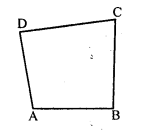(a) no three points of them are collinear
(b) the line segments do not intersect except at their ends points.
(ii) Convex quadrilateral: A quadrilateral is called a convex quadrilateral of the line containing any side of the quadrilateral has the remaining vertices on the same side of it. In the figure, quadrilateral ABCD is a convex quadrilateral.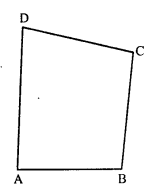Question 2.
In a quadrilateral, define each of the following:
(i) Sides
(ii) Vertices
(iii) Angles
(iv) Diagonals
(vii) Opposite sides
(viii) Opposite angles
(ix) Interior
(x) Exterior
Solution:
(i) Sides: In a quadrilateral ABCD, form line segments AB, BC, CD and DA are called sides of the quadrilateral.
(ii) Vertices : The ends points are called the vertices of the quadrilateral. Here in the figure, A, B, C and D are its vertices.
(iii) Angles: A quadrilateral has four angles which are at their vertices. In the figure, ∠A, ∠B, ∠C and ∠D are its angles.
(iv) Diagonals: The line segment joining the opposite vertices is called diagonal. A quadrilateral has two diagonals.
(v) Adjacent Angles : The angles having a common arm (side) are called adjacent angles.
(vi) Adjacent sides : If two sides of a quadrilateral have a common end-point, these are called adjacent sides.
(vii) Opposite sides: If two sides do not have a common end-point of a quadrilateral, they are called opposite sides.
(viii) Opposite angles : The angles which are not adjacent are called opposite angles.
(ix) Interior: The region which is surrounded by the sides of the quadrilateral is called its interior.
(x) Exterior : The part of the plane made up by all points as the not enclosed by the quadrilateral, is called its exterior.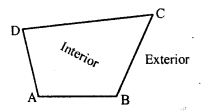Question 3.
Complete each of the following, so as to make a true statement:
(i) A quadrilateral has ………… sides.
(ii) A quadrilateral has ………… angles.
(iii) A quadrilateral has ……….. vertices, no three of which are …………
(iv) A quadrilateral has …………. diagonals.
(v) The number of pairs of adjacent angles of a quadrilateral is ………….
(vi) The number of pairs of opposite angles of a quadrilateral is ……………
(vii) The sum of the angles of a quadrilateral is …………
(viii) A diagonal of a quadrilateral is a line segment that joins two ………. vertices of the quadrilateral.
(ix) The sum of the angles of a quadrilateral is …………. right angles.
(x) The measure of each angle of a convex quadrilateral is …………. 180°.
(xi) In a quadrilateral the point of intersection of the diagonals lies in ………….. of the quadrilateral.
(xii) A point is in the interior of a convex quadrilateral, if it is in the ……….. of its two opposite angles.
(xiii) A quadrilateral is convex if for each side, the remaining …………. lie on the same side of the line containing the side.
Solution:
(i) A quadrilateral has four sides.
(a) A quadrilateral has four angles.
(iii) A quadrilateral has four vertices, no three of which are collinear .
(iv) A quadrilateral has two diagonals.
(v) The number of pairs of adjacent angles of a quadrilateral is four .
(vi) The number of pairs of opposite angles ot a quadrilateral is two.
(vii) The sum of the angles of a quadrilateral is 360°.
(viii) A diagonal of a quadrilateral is a line segment that join two opposite vertices of the quadrilateral.
(ix) The sum of the angles of a quadrilateral is 4 right angles.
(x) The measure of each angle of a convex quadrilateral is less than 180°.
(xi) In a quadrilateral the point of intersection of the diagonals lies in interior of the quadrilateral.
(xii) A point is in the interior of a convex quadrilateral, if it is in the interior of its two opposite angles.
(xiii) A quadrilateral is convex if for each side, the remaining vertices lie on the same side of the line containing the side.

Question 4.
In the figure, ABCD is a quadrilateral.
(i) Name a pair of adjacent sides.
(ii) Name a pair of opposite sides.
(iii) How many pairs of adjacent sides are there?
(iv) How many pairs of Opposite sides are there ?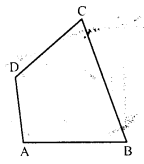(v) Name a pair of adjacent angles.
(vi) Name a pair of opposite angles.
(vii) How many pairs of adjacent angles are there ?
(viii) How many pairs of opposite angles are there ?
Solution:
In the figure, ABCD is a quadrilateral
(i) Pairs of adjacent sides are AB, BC, BC, CD, CD, DA, DA, AB.
(ii) Pairs of opposite sides are AB and CD; BC and AD.
(iii) There are four pairs of adjacent sides.
(iv) There are two pairs of opposite sides.
(v) Pairs of adjacent angles are ∠A, ∠B; ∠B, ∠C; ∠C, ∠D; ∠D, ∠A.
(vi) Pairs of opposite angles are ∠A and ∠C; ∠B and ∠D.
(vii) There are four pairs of adjacent angles.
(viii) There are two pairs of opposite angles.

Question 5.
The angles of a quadrilateral are 110°, 72°, 55° and x°. Find the value of x.
Solution:
Sum of four angles of quadrilateral is 360°
110° + 12° + 55° + x° = 360°
⇒ 237° + x° = 360°
⇒ x° = 360° – 237° = 123°
x = 123°

Question 6.
The three angles of a quadrilateral are respectively equal to 110°, 50° and 40°. Find its fourth angle.
Solution:
The sum of four angles of a quadrilateral = 360°
Three angles are 110°, 50° and 40°
Let fourth angle = x
Then 110° + 50° + 40° + x° = 360°
⇒ 200° + x° = 360°
⇒ x = 360° – 200° = 160°
x = 160°

Question 7.
A quadrilateral has three acute angles each measures 80°. What is the measure of fourth angle ?
Solution:
Sum of four angles of a quadrilateral = 360°
Sum of three angles having each angle equal to 80° = 80° x 3 = 240°
Let fourth angle = x
Then 240° + x = 360°
⇒ x° = 360° – 240°
⇒ x° = 120°
Fourth angle = 120°

Question 8.
A quadrilateral has all its four angles of the same measure. What is the measure of each ?
Solution:
Let each equal angle of a quadrilateral = x
4x° = 360°
⇒ x° = $$\frac { 360 }{ 4 }$$ = 90°
Each angle will be = 90°

Question 9.
Two angles of a quadrilateral are of measure 65° and the other two angles are equal. What is the measure of each of these two angles ?
Solution:
Measures of two angles each = 65°
Sum of these two angles = 2 x 65°= 130°
But sum of four angles of a quadrilateral = 360°
Sum of the remaining two angles = 360° – 130° = 230°
But these are equal to each other
Measure of each angle = $$\frac { 230 }{ 2 }$$ = 115°

Question 10.
Three angles of a quadrilateral are equal. Fourth angle is of measure 150°. What is the measure of equal angles ?
Solution:
Sum of four angles of a quadrilateral = 360°
One angle = 150°
Sum of remaining three angles = 360° – 150° = 210°
But these three angles are equal
Measure of each angle = $$\frac { 210 }{ 3 }$$ = 70°

Question 11.
The four angles of a quadrilateral are as 3 : 5 : 7 : 9. Find the angles.
Solution:
Sum of four angles of a quadrilateral = 360°
and ratio in angles = 3 : 5 : 7 : 9
Let first angles = 2x
Then second angle = 5x
third angle = 7x
and fourth angle = 9x
3x + 5x + 7x + 9x = 360°
⇒ 24x = 369°
⇒ x = $$\frac { 360 }{ 24 }$$ = 15°
First angle = 3x = 3 x 15° = 45°
second angle = 5x = 5 x 15° = 75°
third angle = 7x = 7 x 15° = 105°
and fourth angle = 9x = 9 x 15° = 135°

Question 12.
If the sum of the two angles of a quadrilateral is 180°, what is the sum of the remaining two angles ?
Solution:
Sum of four angles of a quadrilateral = 360°
and sum of two angle out of these = 180°
Sum of other two angles will be = 360° – 180° = 180°

Question 13.
In the figure, find the measure of ∠MPN.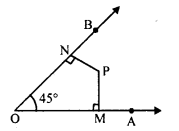Solution:
In the figure, OMPN is a quadrilateral in which
∠O = 45°, ∠M = ∠N = 90° (PM ⊥ OA and PN ⊥ OB)
Let ∠MPN = x°
∠O + ∠M + ∠N + ∠MPN = 360° (Sum of angles of a quadrilateral)
⇒ 45° + 90° + 90° + x° = 360°
⇒ 225° + x° = 360°
⇒ x° = 360° – 225°
⇒x = 135°
∠MPN = 135°

Question 14.
The sides of a quadrilateral are produced in order. What is the sum of the four exterior angles ?
Solution:
The sides of a quadrilateral ABCD are produced in order, forming exterior angles ∠1, ∠2, ∠3 and ∠4.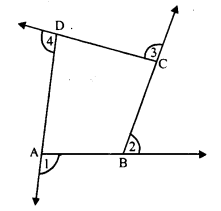Now ∠DAB + ∠1 = 180° (Linear pair) ……(i)
Similarly,
∠ABC + ∠2 = 180°
∠BCD + ∠3 = 180°
and ∠CDA + ∠4 = 180°
∠DAB + ∠1 + ∠ABC + ∠2 + ∠BCD + ∠3 + ∠CDA + ∠4 = 180° + 180° + 180° + 180° = 720°
⇒ ∠DAB + ∠ABC + ∠CDA + ∠ADC + ∠1 + ∠2 + ∠3 + ∠4 = 720°
But ∠DAB + ∠ABC + ∠CDA + ∠ADB = 360° (Sum of angles of a quadrilateral)
360° + ∠1 + ∠2 + ∠3 + ∠4 = 720°
⇒ ∠l + ∠2 + ∠3 + ∠4 = 720° – 360° = 360°
Sum of exterior angles = 360°

Question 15.
In the figure, the bisectors of ∠A and ∠B meet at a point P. If ∠C = 100° and ∠D = 50°, find the measure of ∠APB.
Solution: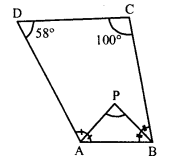∠D = 50°, ∠C = 100°
PA and PB are the bisectors of ∠A and ∠B.
∠A + ∠B + ∠C + ∠D = 360° (Sum of angles of a quadrilateral)
⇒ ∠A + ∠B + 100° + 50° = 360°
⇒ ∠A + ∠B + 150° = 360°’
⇒ ∠A + ∠B = 360° – 150° = 210°
and $$\frac { 1 }{ 2 }$$ ∠A + $$\frac { 1 }{ 2 }$$ ∠B = $$\frac { 210 }{ 2 }$$ = 105°
(PA and PB are bisector of ∠A and ∠B respectively)
∠PAB + ∠PBA = 105°
⇒ ∠PAB + ∠PBA + ∠APB = 180° (Sum of angles of a triangle)
⇒ 105° + ∠APB = 180°
⇒ ∠APB = 180° – 105° = 75°
∠APB = 75°

Question 16.
In a quadrilateral ABCD, the angles A, B, C and D are in the ratio 1 : 2 : 4 : 5. Find the measure of each angle of the quadrilateral.
Solution:
Sum of angles A, B, C and D of a quadrilateral = 360°
i.e. ∠A + ∠B + ∠C + ∠D = 360°
But ∠A = ∠B = ∠C = ∠D = 1 : 2 : 4 : 5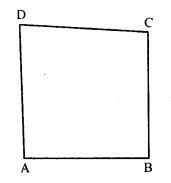Let ∠A = x,
Then ∠B = 2x
∠C = 4x
∠D = 5x
x + 2x + 4x + 5x = 360°
⇒ 12x = 360°
⇒ x = $$\frac { 360 }{ 12 }$$ = 30°
∠A = x = 30°
∠B = 2x = 2 x 30° = 60°
∠C = 4x = 4 x 30° = 120°
∠D = 5A = 5 x 30° = 150°

Question 17.
In a quadrilateral ABCD, CO and DO are the bisectors of ∠C and ∠D respectively. Prove that ∠COD = $$\frac { 1 }{ 2 }$$ (∠A + ∠B).
Solution: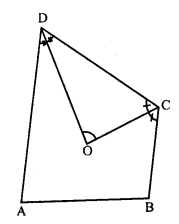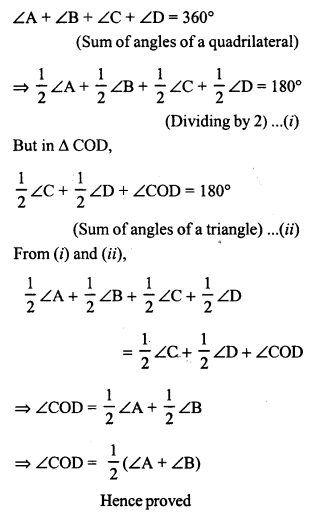Question 18.
Find the number of sides of a regular polygon when each of its angles has a measures of
(i) 160°
(ii) 135°
(iii) 175°
(iv) 162°
(v) 150°.
Solution:
In a n-sided regular polygon, each angle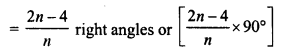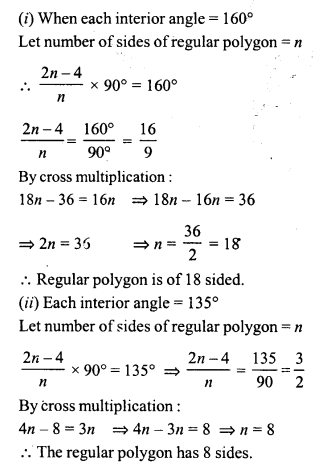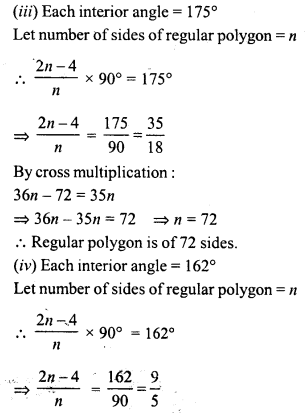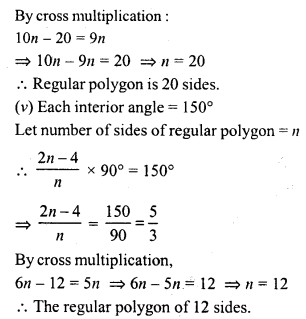Question 19.
Find the number of degrees in each exterior angle of a regular pentagon.
Solution:
In a pentagon or a polygon, sum of exterior angles formed by producing the sides in order, is four right angles or 360°
Each exterior angle = $$\frac { 360 }{ 5 }$$ = 72°

Question 20.
The measure of angles of a hexagon are x°, (x – 5)° (x – 5)°, (2x – 5)°, (2x – 5)°, (2x + 20)°. Find the value of x.
Solution:
We know that the sum of interior angels of a hexagon = 720° (180° x 4)
⇒ x + x – 5 + x – 5 + 2x – 5 + 2x – 5 + 2x + 20 = 720°
⇒ 9x – 20 + 20 = 720
⇒ 9x = 720
⇒ x = $$\frac { 720 }{ 9 }$$ = 80°
x = 80°

Question 21.
In a convex hexagon, prove that the sum of all interior angles is equal to twice the sum of its exterior angles formed by producing the sides in the same order.
Solution:
In a convex hexagon ABCDEF, its sides AB, BG, CD, DE, EF and FA are produced in order forming exterior angles a, b, c, d, e, f∠a + ∠b + ∠c + ∠d + ∠e + ∠f = 4 right angles (By definition)
By joining AC, AD, and AE, 4 triangles ABC, ACD, ADE and AEF are formed
In ∆ABC,
∠1 + ∠2 + ∠3 = 180° = 2 right angle (Sum of angles of a triangle) …… (i)
Similarly,
In ∆ACD,
∠4 +∠5 + ∠6 = 180° = 2 right angles
∠1 + ∠8 + ∠9 = 2 right angles …(iii)
In ∆AEF,
∠10 + ∠11 + ∠12 = 2 right angles …(iv)
Joining (i), (ii), (iii) and (iv)
∠1 + ∠2 + ∠3 + ∠4 + ∠5 + ∠6 + ∠7 + ∠8 + ∠9 + ∠10 + ∠11 + ∠12 = 8 right angles
⇒ ∠2 + ∠3 + ∠5 + ∠6 + ∠8 + ∠9 + ∠11 + ∠12 + ∠1 + ∠4 + ∠7 + ∠10 = 8 right angles
⇒ ∠B + ∠C + ∠D + ∠E +∠F + ∠A = 8 right angles
⇒ ∠A + ∠B + ∠C + ∠D + ∠E + ∠F = 2 (∠a + ∠b + ∠c + ∠d + ∠e + ∠f)
Sum of all interior angles = 2(the sum of exterior angles)
Hence proved.

Question 22.
The sum of the interior angles of a polygon is three times the sum of its exterior angles. Determine the number of sides of the polygon.
Solution:
Let number of sides of a regular polygon = n
Each interior angle = $$\frac { 2n – 4 }{ n }$$ right angles
Sum of all interior angles = $$\frac { 2n – 4 }{ n }$$ x n
right angles = (2n – 4) right angles
But sum of exterior angles = 4 right angles
According to the condition,
(2n – 4) = 3 x 4 (in right angles)
⇒ 2n – 4 = 12
⇒ 2n = 12 + 4 = 16
⇒ n = 8
Number of sides of the polygon = 8

Question 23.
Determine the number of sides of a polygon whose exterior and interior angles are in the ratio 1 : 5.
Solution:
Ratio in exterior angle and interior angles of a regular polygon = 1 : 5
But sum of interior and exterior angles = 180° (Linear pair)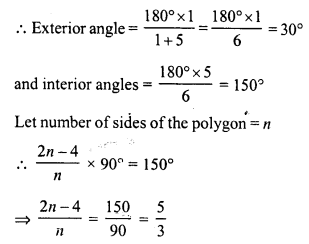By cross multiplication:
6n – 12 = 5n
⇒ 6n – 5n = 12
⇒ n = 12
Number of sides of polygon is 12

Question 24.
PQRSTU is a regular hexagon. Determine each angle of ∆PQT.
Solution:
In regular hexagon, PQRSTU, diagonals PT and QT are joined.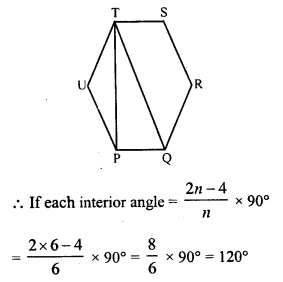In ∆PUT, PU = UT
∠UPT = ∠UTP
But ∠UPT + ∠UTP = 180° – ∠U = 180° – 120° = 60°
∠UPT = ∠UTP = 30°
∠TPQ = 120° – 30° = 90° (QT is diagonal which bisect ∠Q and ∠T)
∠PQT = $$\frac { 120 }{ 2 }$$ = 60°
Now in ∆PQT,
∠TPQ + ∠PQT + ∠PTQ = 180° (Sum of angles of a triangle)
⇒ 90° + 60° + ∠PTQ = 180°
⇒ 150° + ∠PTQ = 180°
⇒ ∠PTQ = 180° – 150° = 30°
Hence in ∆PQT,
∠P = 90°, ∠Q = 60° and ∠T = 30°

Hope given RD Sharma Class 8 Solutions Chapter 16 Understanding Shapes II Ex 16.1 are helpful to complete your math homework.

If you have any doubts, please comment below. Learn Insta try to provide online math tutoring for you.